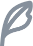reactive函数与ref函数的使用区别，以及如何修改响应式数据。

# 如何定义响应式数据🎞️

## reactive函数简介

1. reactive函数只对对象类型有效对象、数组和 Map、Set 这样的集合类型
2. 对 string、number 和 boolean 这样的原始类型无效
``````// 创建响应式数组
const arr = reactive([1, 2, 3]);

// 创建响应式对象
const obj = reactive({
name: 'John',
age: 30,
});

// 创建响应式Set
const set = reactive(new Set([1, 2, 3]));

// 创建响应式Map
const map = reactive(new Map([['key1', 'value1'], ['key2', 'value2']]));

// 对创建的响应式数据进行修改
arr.push(4);
obj.age++;
map.set('key3', 'value3');

``````

### 错误示例

reactive不能创建基础类型数据

``````const bool = reactive(false)

const number = reactive(2024)

const msg = reactive('hello world')

``````

## ref函数简介

1. 可以使用任何值类型创建响应式数据
2. 将传入参数的值包装为一个带 .value 属性的 ref 对象在模板中会自动解包不需要带上.value
``````<script>
import {ref} from "vue";

// 对象
const refObj = ref({
title:"响应式基础",
content:"reactive和ref"
})

refObj.value.title = "ref介绍"

// 数组
const refArr = ref([1,2,3])
refArr.value++

// 字符串
const refString = ref('hello')
refString.value+='world'

// 数字
const refNumber = ref(2023)
refNumber .value++

// ....Set Map Boolean
</script>

<template>
<h2>{{ refArr }}</h2>
<h2>{{ refString }}</h2>
<h2>{{ refNumber }}</h2>
</template>
``````

## 什么时候用ref，什么时候用reactive

``````// 在使用中

ref({val:1}) 相当于 reactive({value:{val:1} })

ref("hello") 相当于 reactive({value:"hello"})
``````